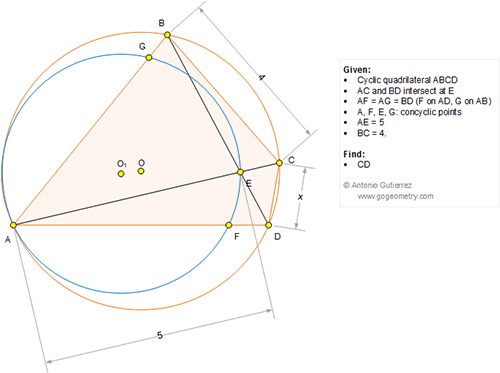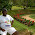## Saturday, September 10, 2016

### Geometry Problem 1258: Cyclic Quadrilateral, Concyclic Points, Measurement

Geometry Problem. Post your solution in the comment box below.
Level: Mathematics Education, High School, Honors Geometry, College.

Click the figure below to view more details of problem 1258.1.Let GE = p, EF = q and FG = r
Let AG = AF = BD = y

Tr.s GEF and BCD are similar so,

p/4 = q/x = r/y .........(1)

Applying Ptolemy to cyclic quad AGEF,
py + qy = 5r and hence from (1).

4r + xr = 5r which yields
x = 1

Sumith Peiris
Moratuwa
Sri Lanka

2.https://goo.gl/photos/ffCv5yAwZMzaTRv7A

Connect FG, EF
Extend BC to H such that CH=CD=x ( see sketch)
We have AG=AF => ∠ (AGF)= ∠ (AFG)= u
Let ∠ (GAE)= w => ∠ (BDC)= ∠ (BAC)= ∠ (GFE)= w
Let ∠ (CAD)= ∠ (DBC)=v
Since ∠ (DCH)= ∠ (BAD) => Isoceles triangles GAF simillar to triangle HCD ( case AAA)=> ∠ (CDH)= u
Triangle AFE congruent to BDH ( case ASA)
So AE= BH=> 5=4+x => x=1

3.Problem 1258
Let the point K on the side AE (no extension) such that AK=BC=4.So KE=1,triangle AFK=triangle BDK(AF=BD,AK=BC,<FAK=<DBC).So CD=KF then <AKF=<BCD=180-<FAG=180-<FKE. So <FAG=<FKE .But <FGA=<GFA=<FEA=<FEK,so <KFE=<KEF(<KFE=180-<FKE-<FEK=
180-<FAG-<FGA=<AFG).So KF=KE=CD=1.
APOSTOLIS MANOLOUDIS 4 HIGH SHCOOL OF KORYDALLOS PIRAEUS GREECE